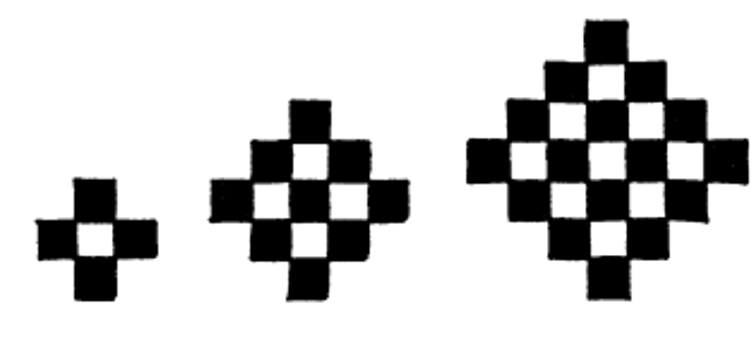Mathematics
Easy

Question

# Lin creates a pattern using the white and black tiles.The number of white tiles present in the next shape of Lin’s pattern is _____.

## 15    16    20    25Hint:

## The correct answer is: 16

### The number of tiles is increasing with the number of shape. The rule used to create the shape is the number of tiles is given by a square. And the number is increasing by 1.The first shape requires 12 = 1 white tiles.The second shape requires 22 = 4 white tiles.The third shape requires 32 = 9 white tiles.So, the next figure will be square of next number that is 4.Therefore, 4th shape requires 42 = 16 white tiles.

We have to be careful about the sequence of the shape. We have to increase the number of tiles in proper order.

### Related Questions to study#### With Turito Foundation.#### Get an Expert Advice From Turito.